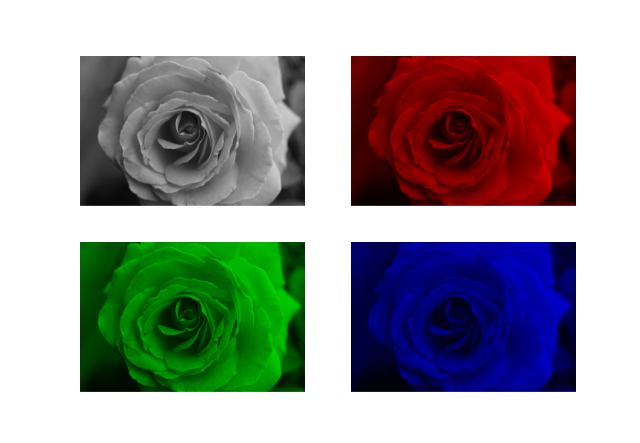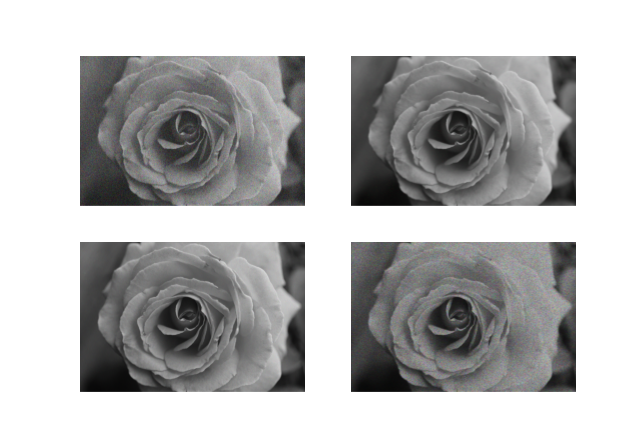# aliquote.org

I always found that Python was great for manipulating strings, or even lists, which is why it remains my language of choice, as opposed to R, for bioinformatics stuff. Needless to say, I hate significant indentation, but other than that I find the language pleasant, and as they said it comes battery included. There’s lot to learn from the base module.

Today, I was trying to make some graphic with Matplotlib and I happened to find some interesting side-effects that we can produce using imshow() alone.

import matplotlib.pyplot as plt
import numpy as np

# Credit: https://www.publicdomainpictures.net/pictures/210000/velka/rose-grayscale.jpg

idx = [[1,1,1], [1,0,0], [0,1,0], [0,0,1]]

for i in range(4):
plt.subplot(2, 2, i+1)
plt.imshow(img*idx[i])
plt.axis('off')Other manipulating RGB channels using simple matrix operations, we can also use more advanced techniques like adding blur or low-pass filter effects with OpenCV2. However, using Numpy alone we can also add some salt and pepper-like effect: (Credit: Shubham Pachori)

def noisy(noise_typ, image):
if noise_typ == "gauss":
row, col, ch = image.shape
mean = 0
var = 0.1
sigma = var**0.5
gauss = np.random.normal(mean, sigma, (row, col, ch))
gauss = gauss.reshape(row, col, ch)
noisy = image + gauss
return noisy
elif noise_typ == "s&p":
row, col, ch = image.shape
s_vs_p = 0.5
amount = 0.004
out = np.copy(image)
num_salt = np.ceil(amount * image.size * s_vs_p)
coords = [np.random.randint(0, i - 1, int(num_salt)) for i in image.shape]
out[coords] = 1
num_pepper = np.ceil(amount * image.size * (1.0 - s_vs_p))
coords = [np.random.randint(0, i - 1, int(num_pepper)) for i in image.shape]
out[coords] = 0
return out
elif noise_typ == "poisson":
vals = len(np.unique(image))
vals = 2 ** np.ceil(np.log2(vals))
noisy = np.random.poisson(image * vals) / float(vals)
return noisy
elif noise_typ == "speckle":
row, col, ch = image.shape
gauss = np.random.randn(row, col, ch)
gauss = gauss.reshape(row, col, ch)
noisy = image + image * gauss
return noisy

type = ["gauss", "s&p", "poisson", "speckle"]
for i in range(4):
plt.subplot(2, 2, i+1)
plt.imshow(noisy(type[i], img/255))
plt.axis('off')♪ Magazine • Back to Nature